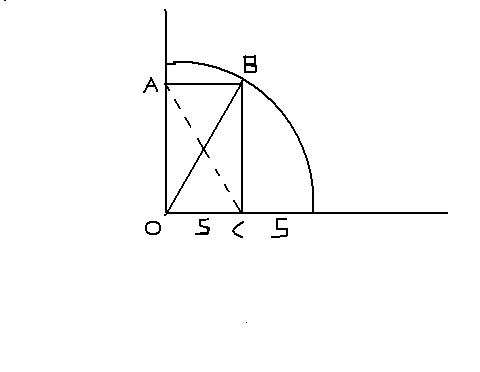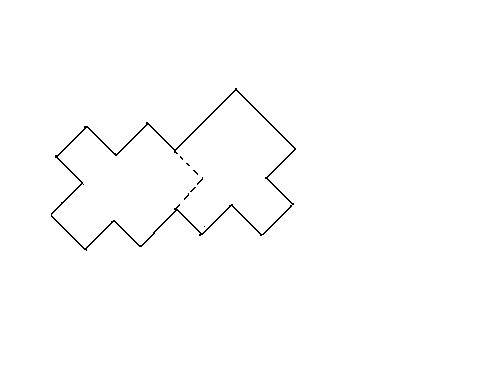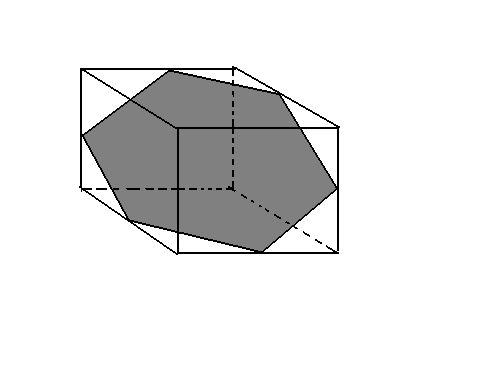Solutions

---------------------------------------------------------------------------------------------------------------------------------------------------------------------------------

1. It is IMPOSSIBLE to cover the chessboard with two opposite corner squares cut off, with 31 dominoes. Here is the proof: The two diagonally opposite corners are of the same colour. Therefore their removal leaves a board with two more squares of one colour than of the other colour. Each domino covers two squares of opposite colour, since only opposite colours are adjacent. After you have covered 60 squares with 30 dominoes, you are left with two uncovered squares of the same colour. These two cannot be adjacent, therefore they cannot be covered by the last domino.

---------------------------------------------------------------------------------------------------------------------------------------------------------------------------------

2. There is NO WAY to reduce the cuts to fewer than six. This is at once apparent when you focus on the fact that a cube has six sides. The saw cuts straight----one side at a time. To cut the one-inch cube at the centre (the one which has no exposed surfaces to start with) must take six passes of the saw.

--------------------------------------------------------------------------------------------------------------------------------------------------------------------------------

3. If we require that the question be answerable by 'yes' or 'no', there are several solutions, all exploiting the same basic gimmick. For example, the logician points to one of the roads and says to the native, 'If I were to ask you if this road leads to the village, would you say 'yes'? ' . The native is forced to give you the right answer, even if he is a liar!!    If the road does lead to the village, the liar would say 'no' to the direct question, but as the question is put in this way, he lies and says he would respond 'yes'. (Of course, a truth-teller says he would respond 'yes' to this question, if the road does lead to the village. Thus the logician can be certain that the road does lead to the village, whether the respondent is a truth-teller or a liar.  On the other hand, if the road actually does not go to the village, the liar is forced in the same way to reply 'no' to the inquirer's question.

A similar question would be, ' If I asked a member of the other tribe whether this road leads to the village, would he say 'yes' ? ' The reader is encouraged to find out the answers to this question by truth-tellers and liars.

--------------------------------------------------------------------------------------------------------------------------------------------------------------------------------

4. You can learn the contents of all three boxes by drawing just ONE marble. The key to the solution is your knowledge that the labels on all three of the boxes are incorrect. You must draw a marble from the box labeled 'black-white'. Assume that the marble drawn is black. You know then that the other marble in this box must be black also, otherwise the label would be correct. Since you have now identified the box containing two black marbles, you can at once tell the contents of the box marked 'white-white': You know it cannot contain two white marbles, because its label has to be wrong; it cannot contain two black, for you have identified that box; therefore it must contain one black and one white. The third box, of course, must then be the one holding two white marbles.

You can solve the puzzle by the same reasoning if the marble you draw from the 'black-white' box happens to be white instead of black.  The reader is encouraged to analyze the solutions after this draw.

-------------------------------------------------------------------------------------------------------------------------------------------------------------------------------

5.  The counterfeit stack can be identified by a single weighing of coins. You take one coin from the first stack, two from the second, three from third and so on to the entire ten coins of the tenth stack. You then weigh the whole sample collection on the pointer scale. The excess weight of this collection, in number of grams, corresponds to the number of the counterfeit stack.

For example, if the group of coins weighs seven grams more than it should, then the counterfeit stack must be the seventh one, from which you took seven coins (each weighing one gram more than a genuine half-dollar).

--------------------------------------------------------------------------------------------------------------------------------------------------------------------------------

6.  Line AC is one diagonal of the rectangle. The other diagonal is clearly the 10-unit radius of the circle. It is clear that the the lengths of AC and BO are the same. And BO is 10 units long. So line AC must be 10 units long.------------------------------------------------------------------------------------------------------------------------------------------------------------------------------

7. Writing a three-digit number twice is the same as multiplying it by 1001. This number has the factors 7, 11, 13, so writing the chosen number twice is equivalent to multiplying the number by 7, 11, 13. Naturally, when the product is successively divided by these same three numbers, the final remainder will be the original number.

Of course, this classical trick is most effective when a single spectator uses a calculator to perform his divisions while your back is tured, only to see his originally chosen number mysteriously (to him!) appear in the readout after the final division.

--------------------------------------------------------------------------------------------------------------------------------------------------------------------------------

8. This problem can be solved quickly by reasoning adroitly as follows: The problem would not be given unless it has a unique solution. If it has a unique solution, the volume must be a constant which could hold even when the hole is reduced to zero radius. Therefore the residue must equal the volume of a sphere with a diameter if six inches. So the volume of a sphere (recall that the formula is volume=4(pi)r^3 /3 where r is radius ) is 36pi.

--------------------------------------------------------------------------------------------------------------------------------------------------------------------------------

9. Twelve matches can be used to form a right triangle with sides of three, four and five units, as shown below. This triangle will have an area of six square units. By altering the position of three matches as shown in the second picture below, we remove two square units, leaving the polygon with an area of four.

This solution is the one to be found in many puzzles books, there are many other solutions.--------------------------------------------------------------------------------------------------------------------------------------------------------------------------------

10. Regardless of how much wine is in one beaker and how much water is in the other, and regardless of how much liquid is transferred back and forth at each step (provided it is not all of the liquid in one beaker), it is IMPOSSIBLE to reach a point at which the percentage of wine in each mixture is the same.

This can be shown by a simple inductive argument. If beaker A contains a higher concentration of wine than beaker B, then a transfer from A to B will leave A with the higher concentration. Similarly a transfer from B to A ----from a weaker to a stronger mixture----is sure to leave B weaker. Since every transfer is one of these two cases, it follows that beaker A must always contain a mixture with a higher percentage of wine than B. The only way to equalize the concentrations is by pouring all of one beaker into the other.

-------------------------------------------------------------------------------------------------------------------------------------------------------------------------------

11. To determine the value of Brown's check, let x stands for the dollars and y for the cents. The problem can now be expressed by the following equation:

100y+x-5 = 2(100x+y)

This reduces to 98y-199x=5, a Diophantine equation with an infinite number of integral solutions. A solution by the standard method of continued fractions gives us the lowest values in positive integers:

x=31, y=63,

making Brown's check \$31.63.  This is a unique answer to the problem because the next lowest values are:

x=129, y=262,

which fails to meet the requirement that y be less than 100.

REMARK: There is a much simpler approach. Note that after buying his newspaper, Brown has left 2x+2y. The change that he has left, from the x cents given him by the cashier, will be x-5.

We know that y is less than 100, but we do not know yet whether it is less than 50 cents. If it is less than 59 cents, we can write the following equations:

2x=y

2y=x-5

If y is 50 cents or more, then Brown will be left with an amount of cents (2y) that is a dollar or more. We therefore have to modify the above equations by taking 100 from 2y and adding 1 to 2x. The equations become:

2x+1=y

2y-100=x-5

Each set of simultaneous equations is easily solved. The first set gives x a minus value, which is ruled out. The second set gives the correct values.

--------------------------------------------------------------------------------------------------------------------------------------------------------------------------------

12. The assumption that the 'lady' is Jean Brown quickly leads to a contradiction. Her opening remark brings forth a reply from the person with black hair, therefore Brown's hair cannot be black. It also cannot be crown, for then it would match her name. Therefore it must be white. This leaves brown for the color of Black's hair and black for White's hair. But a statement by the person with black hair prompts an exclamation from White, so they cannot be the same person.

It is necessary to assume, therefore, that Jean Brown is a man. White's hair can't be white, for then it would match his or her name, nor can it be black because he (or she) replies to the black-haired person. Therefore it must be brown. If the lady's hair isn't brown, then White is not a lady. Brown is a man, so Black must be a lady. Her hair cannot be black or brown, so she must be a platinum blonde.

----------------------------------------------------------------------------------------------------------------------------------------------------------------------------------

13. TWO weighings will do the job.

Divide the nine balls into three sets of triplets. Weigh one triplet against another. If a pan goes down you know the heavy ball is among the three on than pan. Pick any two of these balls and weigh one against the other. If one side goes down, you have found the ball. If they balance, the heavy ball must be the one you put aside. In either case, you have found the odd ball in two weighings.

Suppose the two triplets balance on the first weighing. You know then that the heavy ball is in the remaining triplet. As described above, the heavier ball of this triplet is easily identified by weighing any ball of the triplet against any other.

-----------------------------------------------------------------------------------------------------------------------------------------------------------------------------------

14. The correct cut is like this:----------------------------------------------------------------------------------------------------------------------------------------------------------------------------------

15. Paul wins again. In the first race he ran 100 yards in the time it took for Peter to run 90 yards. Therefore, in the second race, after Peter has gone 90 yards, Paul will have gone 100 yards, so he will be alongside him. Both will have 10 more yards to go.

Since Paul is the faster runner, he will finish the race before Peter.

----------------------------------------------------------------------------------------------------------------------------------------------------------------------------------

16. Here is an 'obvious' proof:

Let ascent and descent be divided between two persons on the same day. They must meet. The readers are encouraged to find out some more proofs themselves.

----------------------------------------------------------------------------------------------------------------------------------------------------------------------------------

17. It is IMPOSSIBLE for the termite to pass once through the 26 outside cubes and end its journey in the center one. There is a pretty simple proof.

This is easily demonstrated by imagining that the cubes alternate in color like the cells of a three-dimensional checkerboard, or the sodium and chlorine atoms in the cubical crystal lattice of ordinary salt. The large cube will then consist of 13 cubes of one color and 14 of the other color.

The termite's path is always through cubes that alternate in color along the way; therefore if the path is to include all 27 cubes, it must begin and end with a cube belonging to the set of 14. The central cube, however, belongs to the 13 set; hence the desired path is impossible.

NOTE: The problem can be generalized as follows: A cube of even order (an even number of cells on the side) has the same number of cells of one color as it has cells of the other color. There is no central cube but complete paths may start on any cell and end on any cell of opposite color. A cube of odd order has one more cell of one color than the other, so a complete path must begin and end on the color that is used for the larger set.  In odd-order cubes of orders, 3, 7, 11, 15, 19... the central cell belongs to the smaller set, so it cannot be the end of any complete path.  In odd-order cubes of 1, 5, 9, 13, 17... the central cell belongs to the larger set, so it can be the end of any path that starts on a cell of the same color. No closed path, going through every unit cube, is possible on any odd-order cube because of the extra cube of one color.

Many two-dimensional puzzles can be solved quickly by similar 'parity checks'. For example, it is not possible for a rook to start at one corner of a chessboard and follow a path that carries it once through every square and ends on the square at the diagonally opposite color.

-----------------------------------------------------------------------------------------------------------------------------------------------------------------------------------

18. A cube. cut in half by plane that passes through the midpoints of six sides as shown below, produces a cross section that is a regular hexagon. If the cube is an inch on the side, the side of the hexagon is 1/(square root of 2) .To cut a torus so that the cross section consists of two intersecting circles, the plane must pass through the centre and be tangent to the torus above and below, as shown below. If the torus and hole have diameters of three inches and one inch, each circle of the section will clearly have a diameter of two inches.------------------------------------------------------------------------------------------------------------------------------------------------------------------------------